# CBSE Solutions for Class 10 Maths

#### Select CBSE Solutions for class 10 Subject & Chapters Wise :

The decimal expansion of the rational number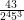will terminate after how many places of decimals? (2013)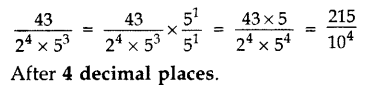Write the decimal form of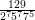Non-terminating non-repeating.

Find the largest number that will divide 398, 436 and 542 leaving remainders 7, 11, and 15 respectively.

Algorithm
398 – 7 = 391, 436 – 11 = 425, 542 – 15 = 527
HCF of 391, 425, 527 = 17

Express 98 as a product of its primes.

2 × 72

If the HCF of 408 and 1032 is expressible in the form 1032 × 2 + 408 × p, then find the value of p.

HCF of 408 and 1032 is 24.
1032 × 2 + 408 × (p) = 24
408p = 24 – 2064
p = -5

HCF and LCM of two numbers is 9 and 459 respectively. If one of the numbers is 27, find the other number. (2012)

We know,
1st number × 2nd number = HCF × LCM
⇒ 27 × 2nd number = 9 × 459
⇒ 2nd number =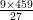= 153

Find HCF and LCM of 13 and 17 by prime factorisation method. (2013)

13 = 1 × 13; 17 = 1 × 17
HCF = 1 and LCM = 13 × 17 = 221

Find LCM of numbers whose prime factorisation are expressible as 3 × 5and 3× 7(2014)

LCM (3 × 52, 32 × 72) = 32 × 52 × 72 = 9 × 25 × 49 = 11025

Find the LCM of 96 and 360 by using fundamental theorem of arithmetic. (2012)

96 = 25 × 3
360 = 23 × 32 × 5
LCM = 25 × 32 × 5 = 32 × 9 × 5 = 1440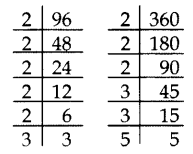Find the HCF (865, 255) using Euclid’s division lemma. (2013)

865 > 255
865 = 255 × 3 + 100
255 = 100 × 2 + 55
100 = 55 × 1 + 45
55 = 45 × 1 + 10
45 = 10 × 4 + 5
10 = 5 × 2 + 0
The remainder is 0.
HCF = 5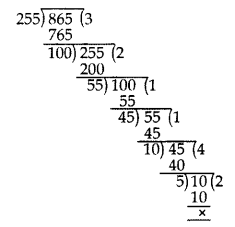Prove that √5 is irrational and hence show that 3 + √5 is also irrational. (2012)

Let us assume, to the contrary, that √5 is rational.
So, we can find integers p and q (q ≠ 0), such that
√5 =, where p and q are coprime.
Squaring both sides, we get
5 =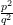5q2 = p2 …(i)
5 divides p2
5 divides p
So, let p = 5r
Putting the value of p in (i), we get
5q2 = (5r)2
5q= 25r2
q2 = 5r2
5 divides q2
5 divides q
So, p and q have atleast 5 as a common factor.
But this contradicts the fact that p and q have no common factor.
So, our assumption is wrong, is irrational.
√5 is irrational, 3 is a rational number.
So, we conclude that 3 + √5 is irrational.

Prove that 3 + 2√3 is an irrational number. (2014)

Let us assume to the contrary, that 3 + 2√3 is rational.
So that we can find integers a and b (b ≠ 0).
Such that 3 + 2√3 =, where a and b are coprime.
Rearranging the equations, we get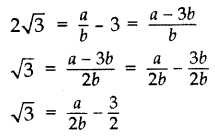Since a and b are integers, we get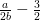is rational and so √3 is rational.
But this contradicts the fact that √3 is irrational.
So we conclude that 3 + 2√3 is irrational.

Three bells toll at intervals of 9, 12, 15 minutes respectively. If they start tolling together, after what time will they next toll together? (2013)

9 = 32, 12 = 22 × 3, 15 = 3 × 5
LCM = 22 × 32 × 5 = 4 × 9 × 5 = 180 minutes or 3 hours
They will next toll together after 3 hours.

Two tankers contain 850 liters and 680 liters of petrol. Find the maximum capacity of a container which can measure the petrol of each tanker in the exact number of times. (2012)

To find the maximum capacity of a container which can measure the petrol of each tanker in the exact number of times, we find the HCF of 850 and 680.
850 = 2 × 52 × 17
680 = 23 × 5 × 17
HCF = 2 × 5 × 17 = 170
Maximum capacity of the container = 170 liters.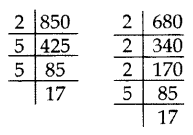​​​​​​​

The length, breadth, and height of a room are 8 m 50 cm, 6 m 25 cm and 4 m 75 cm respectively. Find the length of the longest rod that can measure the dimensions of the room exactly. (2015)

To find the length of the longest rod that can measure the dimensions of the room exactly, we have to find HCF.
L, Length = 8 m 50 cm = 850 cm = 21 × 52 × 17
B, Breadth = 6 m 25 cm = 625 cm = 54
H, Height = 4 m 75 cm = 475 cm = 52 × 19
HCF of L, B and H is 52 = 25 cm
Length of the longest rod = 25 cm

 1 Rational No A Root of -1 2 Irrational No. B Rational & Irrational no. 3 Real No. C 1/3 4 Non-Real No. D Square root of 2

1-C , 2-D, 3-B, 4-A

 1 Whole No. A 1/7 2 Natural No B 0,1,2,3…… 3 Integers. C 1,2,3,4…… 4 Fractions D …-2,-1,0,1,2…

1-B, 2-C, 3-D, 4-A

 1 Even No. A 4,6,8,9,12 2 Odd No . B 2,3,5,7,11 3 Prime No. C 1,3,5,7,11 4 Composite No. D 2,4,6,8,10

1-D, 2-C, 3-B, 4-A

 No. H.C.F. 1 6, 12, 15 A 1 2 4, 8, 16 B 3 3 10, 25, 50 C 4 4 2, 3, 4 D 5

1-B,.  2-C, 3-D, 4-A

 No. L.C.M 1 12, 13 A 150 2 100, 15 B 156 3 50, 60 C 66 4 2, 33 D 300

1-B, 2-A, 3-D, 4-C